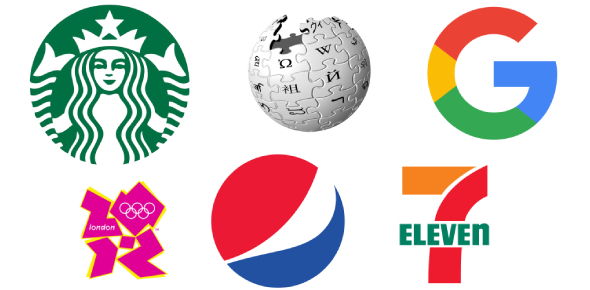# Science - Benchmark III Review (18)

18 Questions | Total Attempts: 207SettingsOverall review of SOLs

• 1.
What is the hardest mineral on earth?
• A.

Gemstone

• B.

Diamond

• C.

Rock

• D.

Granite

• 2.
What type of energy is used when the man "chews" the food?
• A.

Mechanical energy

• B.

Electrical energy

• C.

Nuclear energy

• D.

Light energy

• 3.
What type of energy is lighting?
• A.

Electrical energy

• B.

Mechanical energy

• C.

Light energy

• D.

Nuclear energy

• 4.
What slows down the small object opposed to the elephant?
• A.

Inertai

• B.

Air resistance

• C.

Gravitry

• D.

Vacuum

• 5.
What remains the same on the moon and the earth?
• A.

Weight

• B.

Mass

• C.

Properties

• D.

Gravity

• 6.
What type of instrument is used to measure force?
• A.

Thermometer

• B.

Triple beam balance

• C.

Spring scale

• D.

Meter stick

• 7.
What type of mixture is it when the snow settles to the bottom of the snow globe?
• A.

Colloid

• B.

Suspension

• 8.
What type of change is it when an ice cube melts?
• A.

Chemical change

• B.

Physical change

• C.

Mechanical change

• D.

Weird change

• 9.
What is the process called when an acid and a base combines to form salt and water?
• A.

Salinity

• B.

Water cycle

• C.

Neutralization process

• D.

Life cycle

• 10.
What type of light bulb has a filament instead of argon gas?
• A.

Fluroescent bulb

• B.

Firefly light

• C.

• D.

Neon light

• 11.
What type of molecule is the following?
• A.

Hydrogen

• B.

Helium

• C.

Water

• D.

Oxygen

• 12.
What is the "heaviness" of gold that makes it sink to the bottom of the pan?
• A.

Volume

• B.

Mass

• C.

Density

• D.

Weight

• 13.
What type of energy is the mouse producing?
• A.

Potential energy

• B.

Kinetic energy

• C.

Chemical energy

• D.

Mechanical energy

• 14.
What happens to the water on the sidewalk on a hot day?
• A.

Sublimation

• B.

Condensation

• C.

Evaporation

• D.

Disappears

• 15.
What  type of change is occuring to the toast in a toaster?
• A.

Physical change

• B.

Chemical change

• C.

Reaction

• D.

Net force

• 16.
What does all organic material or once living material contain?
• A.

Oxygen

• B.

Hydrogen

• C.

Carbon

• D.

Cells

• 17.
Which two elements are "chemically" similar?  (remember they must be in the "same" group)
• A.

Argon (Ar) and Neon (Ne

• B.

Iron (Fe) and Chlorine (Cl)

• C.

Sodium (Na) and Gold (Au)

• D.

Carbon (C) and Oxygen (O)

• 18.
Which place is the "mass" the same (not wieght)?
• A.

Saturn

• B.

Earth

• C.

Moon

• D.

Mass is the same in all the above locations.

Related TopicsBack to top